Free Printable Worksheets for Third Grade

## Order of Operations I (Set 1)This is a basic worksheet for practice with simplifying expressions using the correct order of operations. Each problem uses 3 numbers involving addition or subtraction combined with...

## Geometry: Find the Perimeter (Set 2)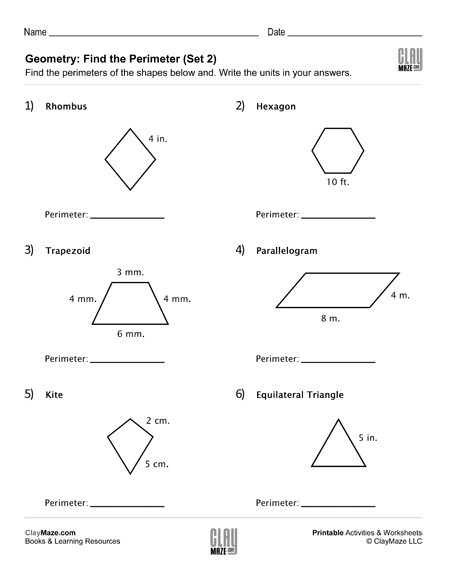Use this free worksheet for practice finding perimeters. The student is asked to find the perimeter of each of the shapes given. This worksheet features a rhombus, a hexagon, a trapezoid, a...

## Geometry: Find the Perimeter (Set 1)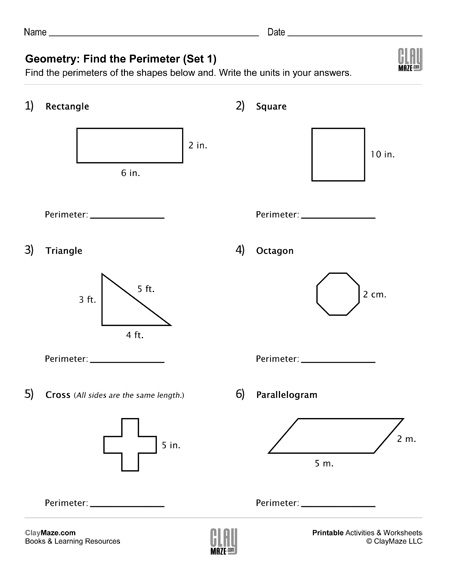Download this geometry worksheet on perimeters. Have the student find the perimeters of each of the shapes shown. This sheet features a rectangle, a square, a triangle, an octagon, a cross and a...

## Long Division Worksheet with Double Digit Divisors (Set 4)Download this long division practice worksheet. It features double digit divisors and no remainders. – 9 problems...

## Long Division Worksheet with Double Digit Divisors (Set 3)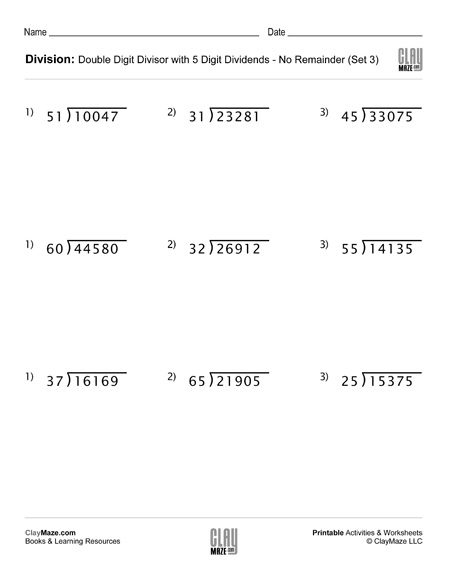Use this worksheet for pracice with long division. The divisors have 2 digits and there are no remainders. – 9 problems...

## Long Division Worksheet with Double Digit Divisors (Set 2)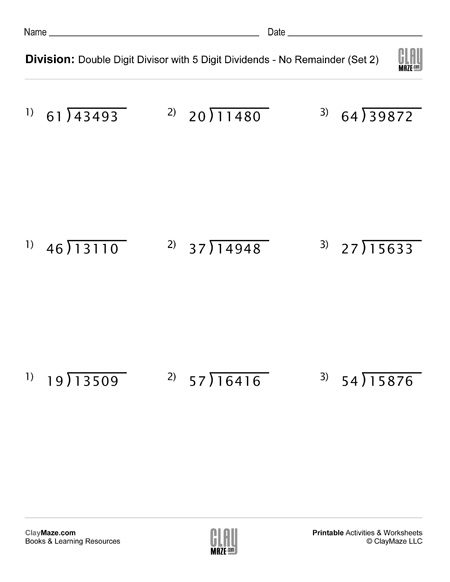This division worksheet is on long division with double digit divisors. There are no remainders. – 9 problems...

## Long Division Worksheet with Double Digit Divisors (Set 1)This worksheet is a set of long division problems with double digit divisors. There are no remainders. – 9 problems...

## Division Worksheet with Single Digit Divisors (Set 4)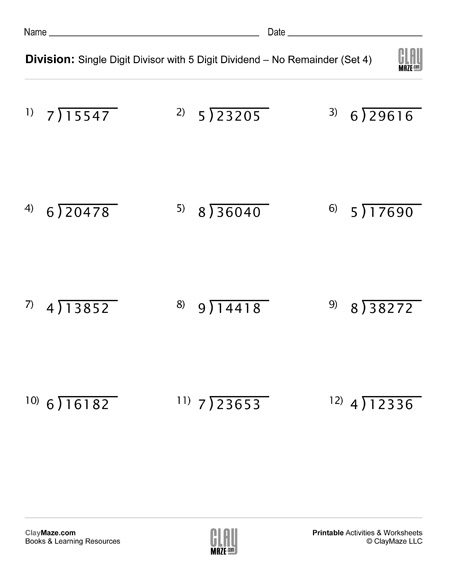Use this worksheet for division practice with single digit divisors. The problems can be solved using either long division or short division. There are no remainders....

## Division Worksheet with Single Digit Divisors (Set 3)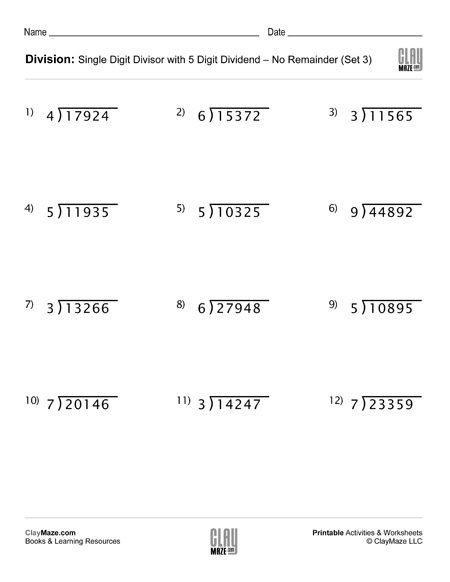This is a worksheet on division practice with a single digit divisor. They can be done using short division or long division. There is plenty of room for either. – No remainders....

## Division Worksheet with Single Digit Divisors (Set 2)This division worksheet can be completed by using either long division or short division. Each problem has a 5-digit dividend and a single digit divisor. – No remainders....

## Division Worksheet with Single Digit Divisors (Set 1)This is a division worksheet with 5 digit dividends and single digit divisors. They can be solved by either short division or long division. There are no remainders....

## Spy Decoder Wheel – Random Symbol Version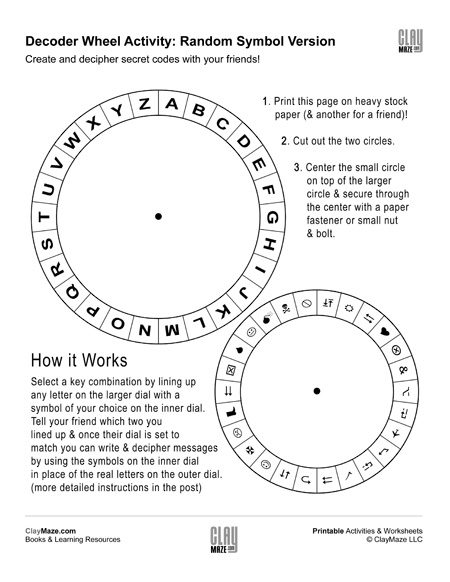This template is the Symbol Version of our Alphabet Decoder Wheel.  There are three decoder wheels in all allowing for the coding and deciphering of secret messages using random alphabet...

## Spy Decoder Wheel – Custom Code VersionThis template is the customizable version of our Random Alphabet Decoder Wheel.  There are three decoder wheels in all allowing for the coding and decoding of secret messages using random...

## Spy Decoder Wheel – Random Alphabet VersionHow the Decoder Works Once you’ve used the instructions on the PDF Download to assemble your decoder you can either share that decoder with a friend or have them make an identical one.  To use it, ...

## Roman Numerals to Arabic Numerals: Higher NumbersRoman numerals free practice worksheet. Convert the Roman numerals to Arabic Numerals. – higher numbers Click here for our Roman numeral reference chart....